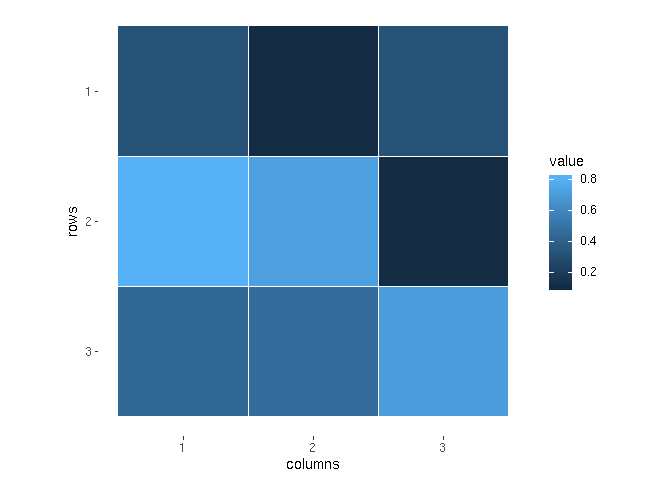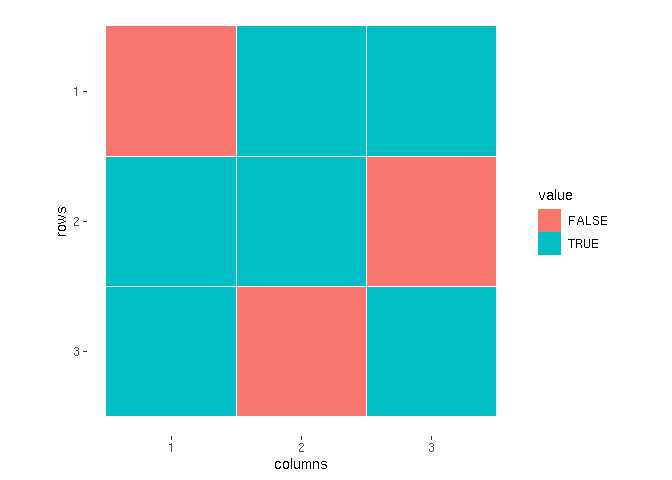Want to share your content on R-bloggers? click here if you have a blog, or here if you don't.`matricks` package in 0.8.2 version has been released on CRAN! In
this post I will present you, what are advantages of using `matricks`
and how you can use it.

### Creating matrices

The main function the package started with is `m`. It’s a smart shortcut
for creating matrices, especially usefull if you want to define a matrix
by enumerating all the elements row-by-row. Typically, if you want to
create a matrix in R, you can do it using `base` function called
`matrix`.

``````matrix(c(3 ,4, 7,
5, 8, 0,
9, 2, 1), nrow = 3, byrow = TRUE)
``````
``````##      [,1] [,2] [,3]
## [1,]    3    4    7
## [2,]    5    8    0
## [3,]    9    2    1
``````

Although it’s a very simple opeartion, the funtion call doesn’t look
tidy. Alternaively, we can use `tibble` with its `frame_matrix`
function, defining column names with formulae first.

``````library(tibble)
frame_matrix(~ c1, ~ c2, ~ c3,
3,    4,    7,
5,    8,    0,
9,    2,    1)
``````
``````##      c1 c2 c3
## [1,]  3  4  7
## [2,]  5  8  0
## [3,]  9  2  1
``````

However, it’s still not a such powerfull tool as `matricks::m` function
is. Let’s see an example.

``````library(matricks)
m(3 ,4, 7|
5, 8, 0|
9, 2, 1)
``````
``````##      [,1] [,2] [,3]
## [1,]    3    4    7
## [2,]    5    8    0
## [3,]    9    2    1
``````

As simple as that! We join following rows using `|` operator. `m`
function is very flexible and offers you much more than before mentioned
ones.

``````m(1:3 | 4, 6, 7 | 2, 1, 4)
``````
``````##      [,1] [,2] [,3]
## [1,]    1    2    3
## [2,]    4    6    7
## [3,]    2    1    4
``````

And here and example with bindig multiple matrices together:

``````mat1   <- diag(1, 3, 3)
mat2  <- antidiag(1, 3, 3) * 3
m(mat1, mat2|
mat2, mat1)
``````
``````##      [,1] [,2] [,3] [,4] [,5] [,6]
## [1,]    1    0    0    0    0    3
## [2,]    0    1    0    0    3    0
## [3,]    0    0    1    3    0    0
## [4,]    0    0    3    1    0    0
## [5,]    0    3    0    0    1    0
## [6,]    3    0    0    0    0    1
``````

By the way, `antidiag` function can be found in the `matricks` package
too.

### Setting & accessing values

These code

``````mat <- matrix(0, 3, 3)
mat[1, 2] <- 0.3
mat[2, 3] <- 7
mat[3, 1] <- 13
mat[2, 2] <- 0.5
mat
``````
``````##      [,1] [,2] [,3]
## [1,]    0  0.3    0
## [2,]    0  0.5    7
## [3,]   13  0.0    0
``````

can be replaced with:

``````mat <- matrix(0, 3, 3)
mat <- set_values(mat,
c(1, 2) ~ 0.3,
c(2, 3) ~ 7,
c(3, 1) ~ 13,
c(2, 2) ~ 0.5)
mat
``````
``````##      [,1] [,2] [,3]
## [1,]    0  0.3    0
## [2,]    0  0.5    7
## [3,]   13  0.0    0
``````

In some cases, traditional way we access a matrix element in `R` may be
inconvenient. Consider situation shown below:

``````sample.matrix <- matrix(1, 3, 3)
matrix.indices <- list(c(1, 1), c(1, 2),
c(1, 3), c(2, 2),
c(3, 1), c(3, 3))

for (idx in matrix.indices) {
sample.matrix[idx, idx] <- sample.matrix[idx, idx] + 2
}

sample.matrix
``````
``````##      [,1] [,2] [,3]
## [1,]    3    3    3
## [2,]    1    3    1
## [3,]    3    1    3
``````

It can be expressed conciser using matrix `at` function.

``````sample.matrix <- matrix(1, 3, 3)
matrix.indices <- list(c(1, 1), c(1, 2),
c(1, 3), c(2, 2),
c(3, 1), c(3, 3))

for (idx in matrix.indices) {
at(sample.matrix, idx) <- at(sample.matrix, idx) + 2
}
sample.matrix
``````
``````##      [,1] [,2] [,3]
## [1,]    3    3    3
## [2,]    1    3    1
## [3,]    3    1    3
``````

### Plotting matrix

`matrix` objects haven’t had good automatized plotting function until
now. Let’s create and plot a sample matrix of random values.

``````rmat <- runifm(3, 3)
print(rmat)
``````
``````##           [,1]      [,2]      [,3]
## [1,] 0.3248890 0.1024049 0.3295454
## [2,] 0.8077164 0.7267801 0.1116789
## [3,] 0.4406909 0.4703106 0.7047498
``````
``````plot(rmat)
``````And here the same using a matrix of random boolean values (`rboolm`).

``````set.seed(7)
rmat <- rboolm(3, 3)
print(rmat)
``````
``````##       [,1]  [,2]  [,3]
## [1,] FALSE  TRUE  TRUE
## [2,]  TRUE  TRUE FALSE
## [3,]  TRUE FALSE  TRUE
``````
``````plot(rmat)
``````### Operators

`matricks` contains a family of operators, which allows you to perform
column-/row-wise operation
vector.

``````mat <- m(1, 2, 3|
4, 5, 6|
7, 8, 9)
mat
``````
``````##      [,1] [,2] [,3]
## [1,]    1    2    3
## [2,]    4    5    6
## [3,]    7    8    9
``````
``````vec <- v(1:3)
vec
``````
``````##      [,1]
## [1,]    1
## [2,]    2
## [3,]    3
``````

If we try to do a column-wise multiplication, we ecounter a problem.

``````mat * vec
``````
``````## Error in mat * vec: niezgodne tablice
``````

We can bypass this error using `%m%` function. It does what we want!

``````mat %m% vec
``````
``````##      [,1] [,2] [,3]
## [1,]    1    2    3
## [2,]    8   10   12
## [3,]   21   24   27
``````

There are also several other operators available.

``````mat %d% vec
``````
``````##          [,1]     [,2] [,3]
## [1,] 1.000000 2.000000    3
## [2,] 2.000000 2.500000    3
## [3,] 2.333333 2.666667    3
``````
``````mat %+% vec
``````
``````##      [,1] [,2] [,3]
## [1,]    2    3    4
## [2,]    6    7    8
## [3,]   10   11   12
``````
``````mat %-% vec
``````
``````##      [,1] [,2] [,3]
## [1,]    0    1    2
## [2,]    2    3    4
## [3,]    4    5    6
``````

I encourage you to familiarize with `matricks`. Visit matrix
documentation